210621 Epaper

 A Section B SectionC Section D Section210620 Epaper

 A Section B SectionC Section D Section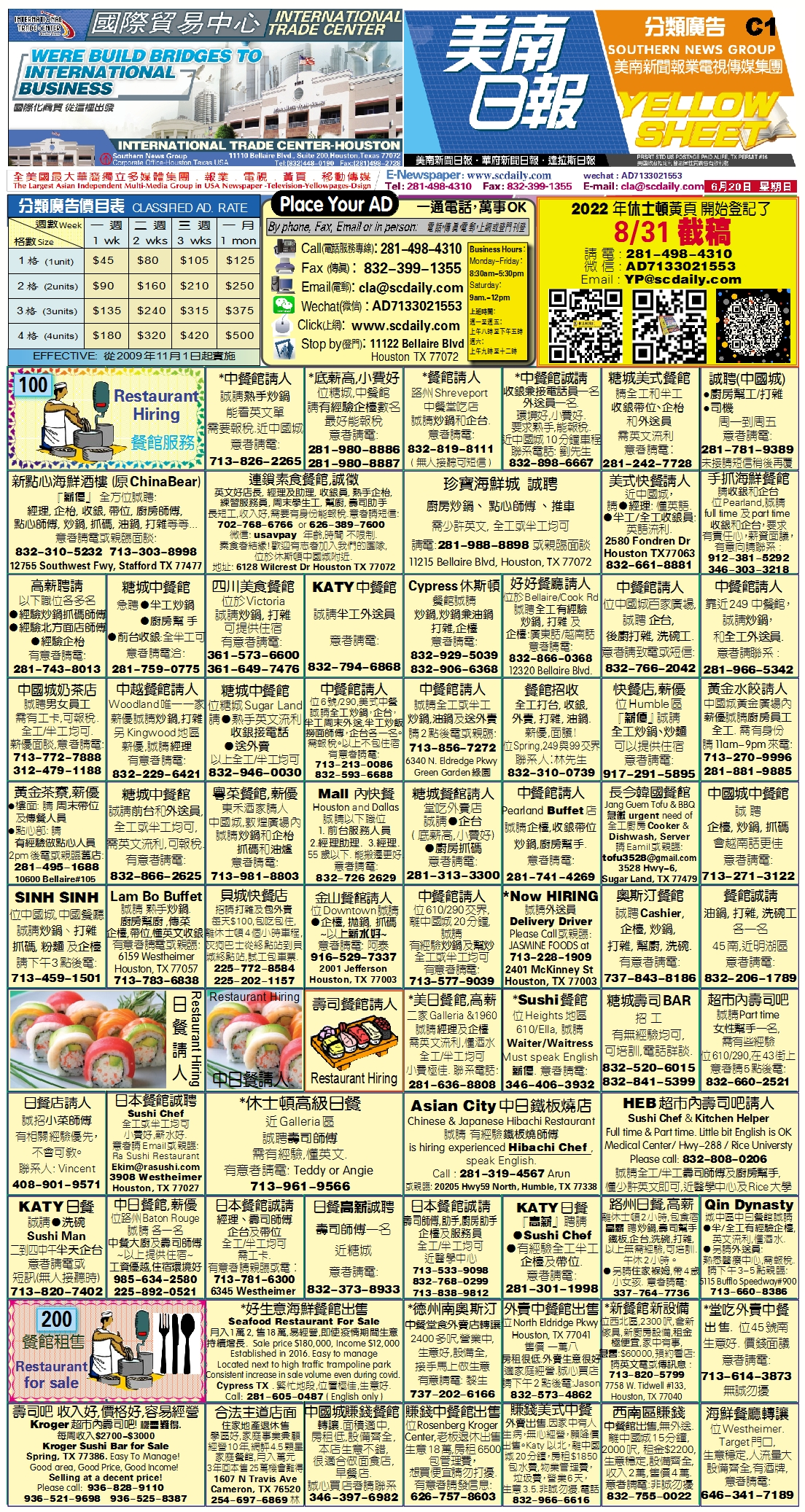210619 Epaper

 A Section B SectionC Section D Section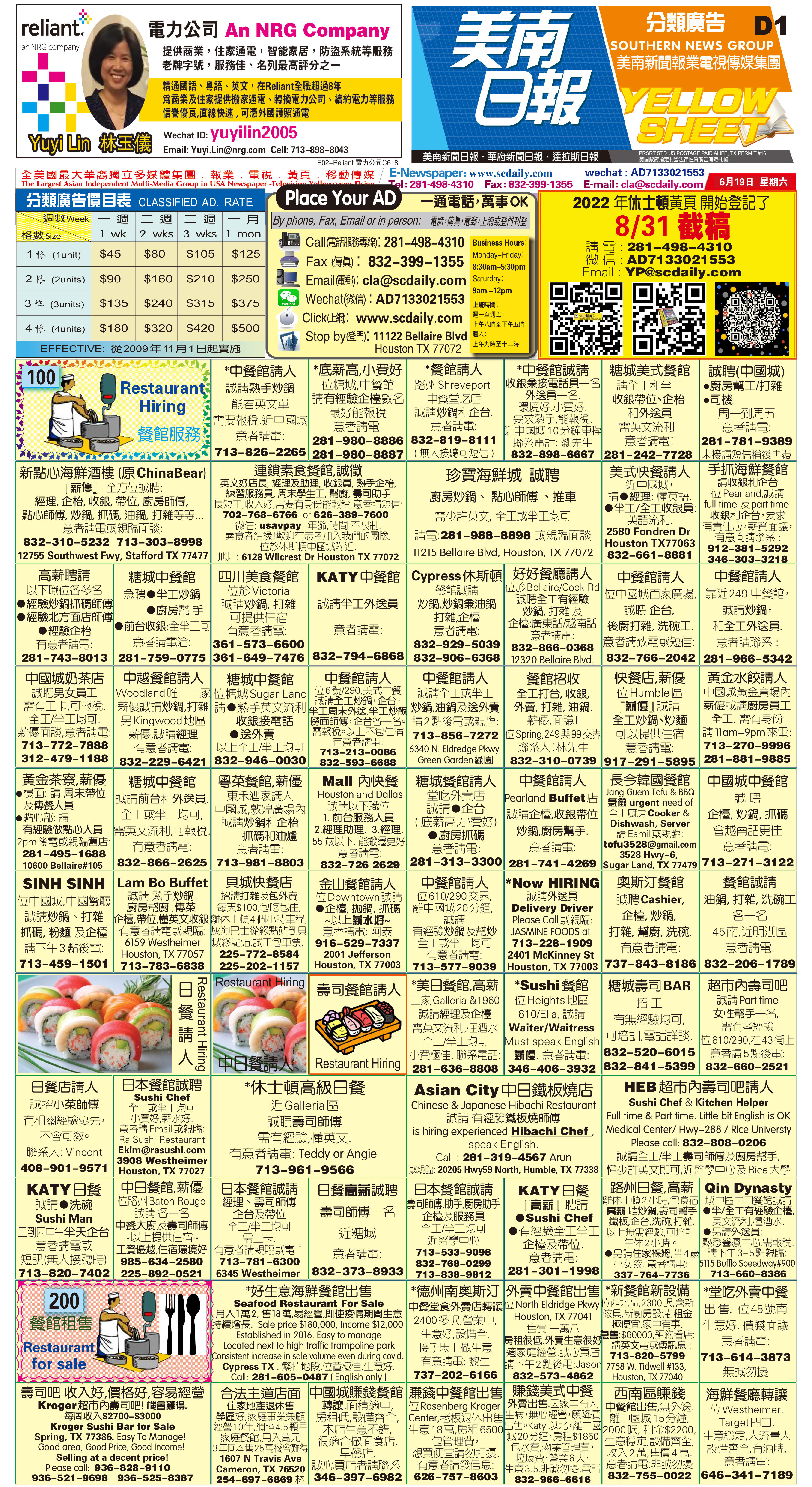210618 Epaper

 A Section B SectionC Section D Section210617 Epaper

 A Section B SectionC Section D Section210616 Epaper

 A Section B SectionC Section D Section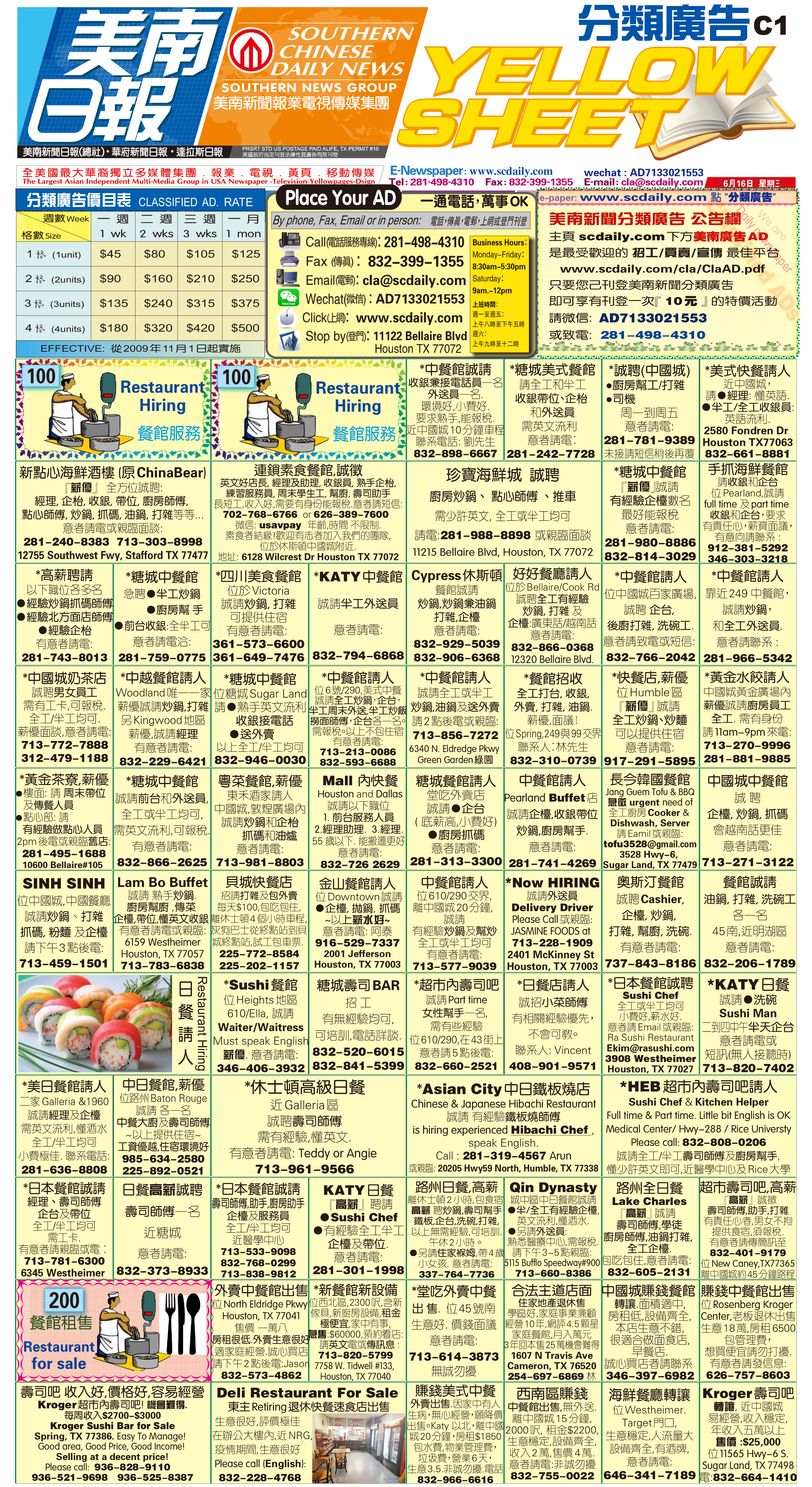210615 Epaper

 A Section B SectionC Section D Section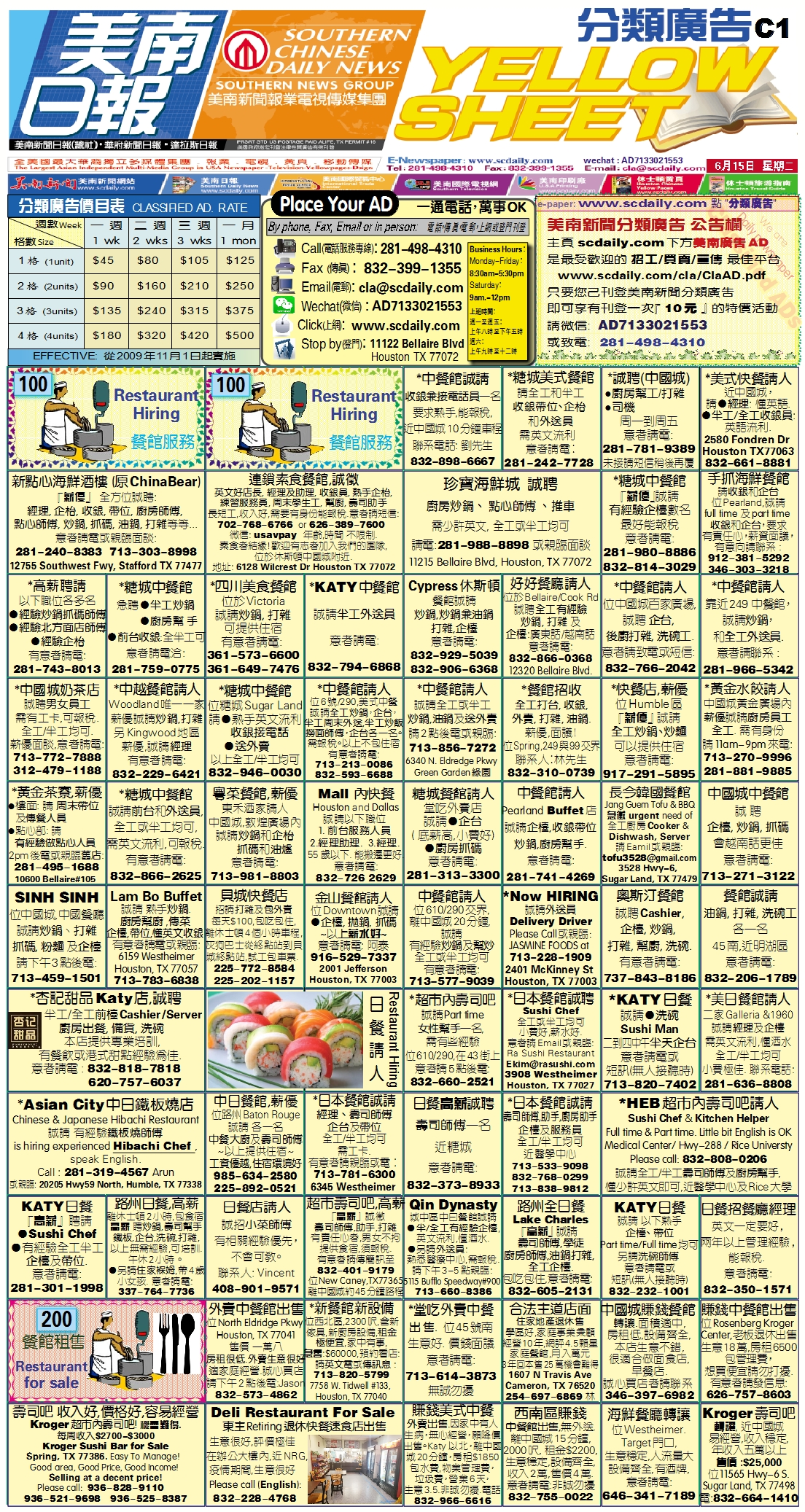210614 Epaper

 A Section B SectionC Section D Section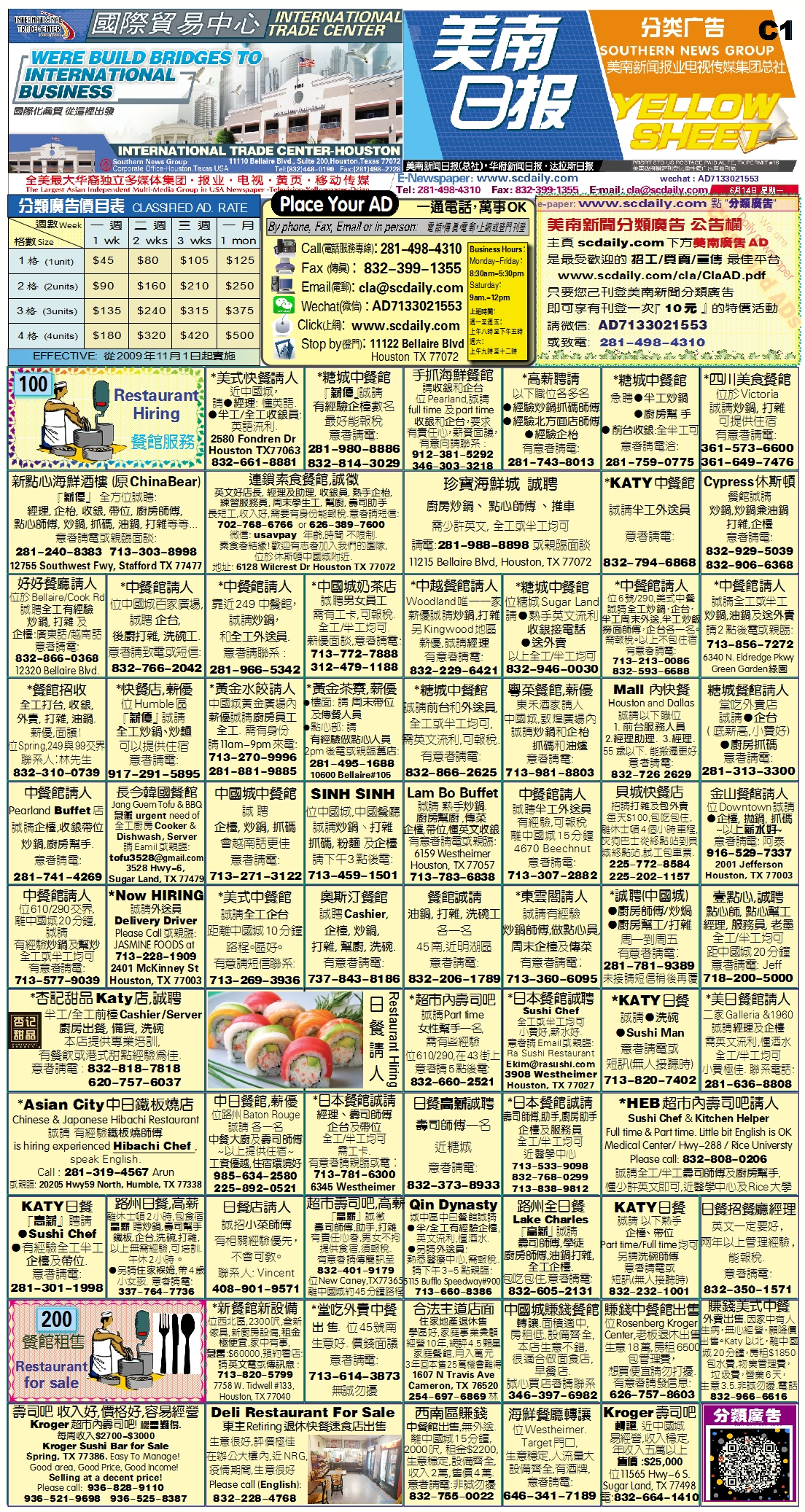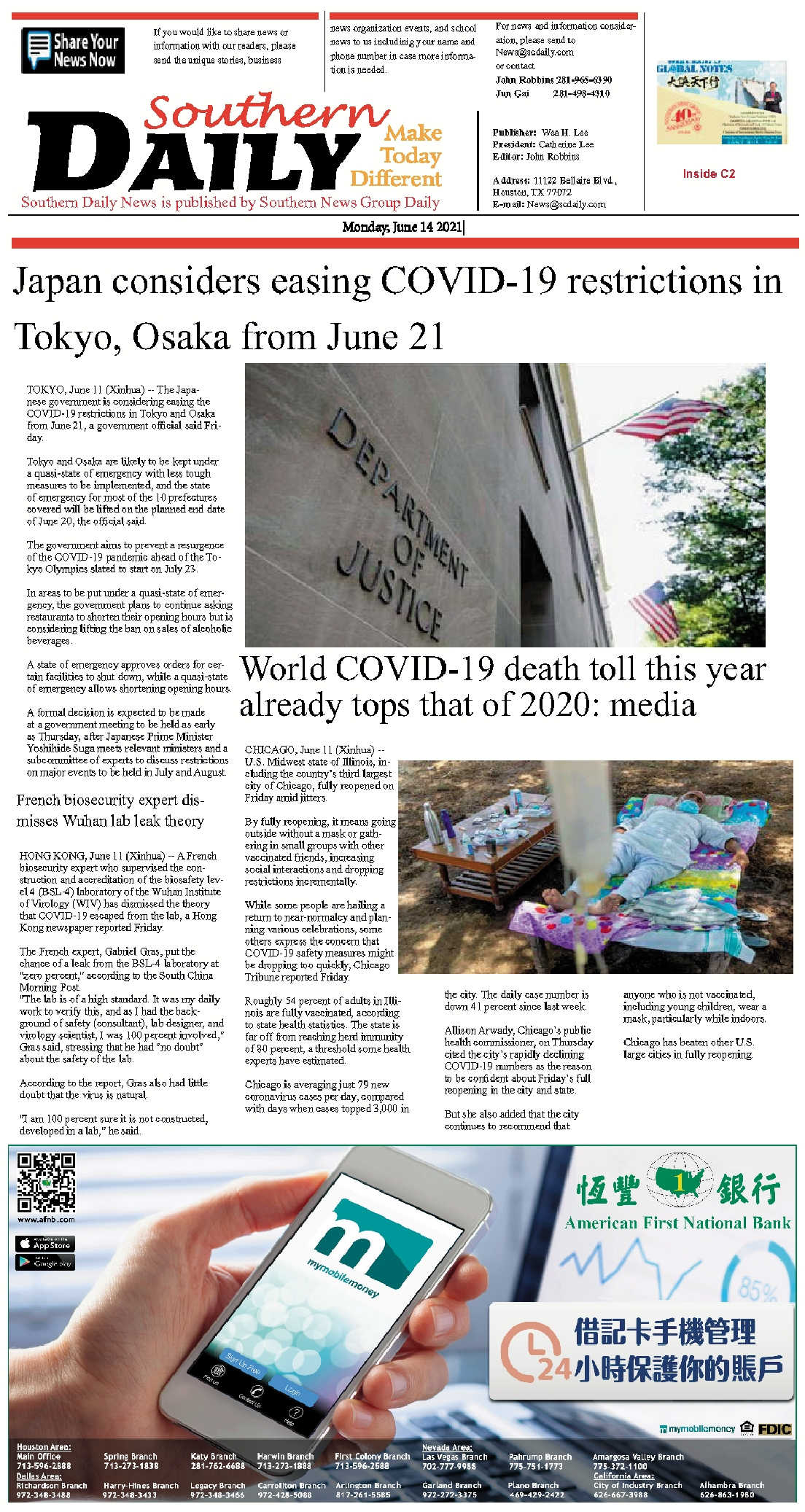210612 Epaper

 A Section B SectionC Section D Section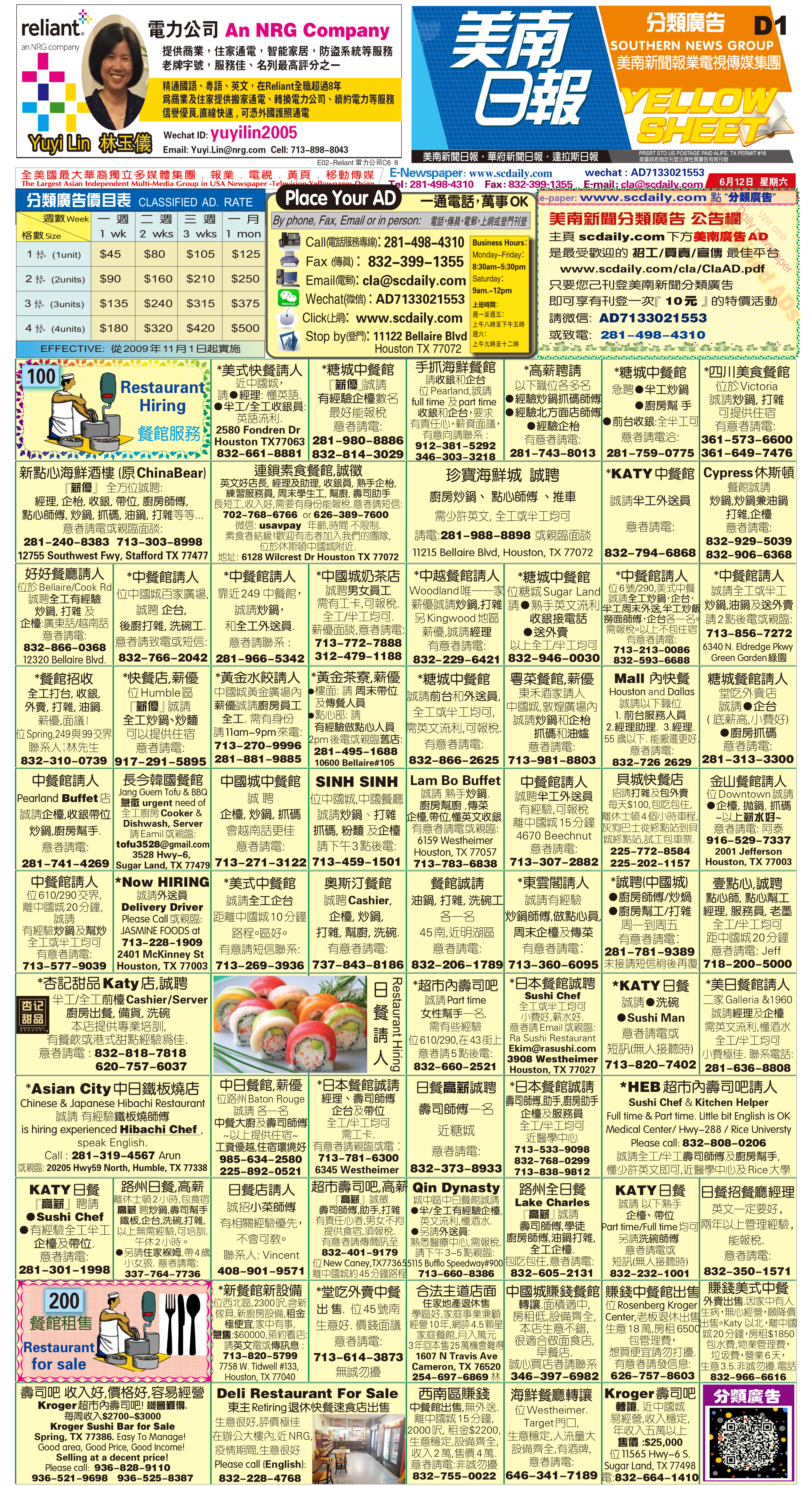210613 Epaper

 A Section B SectionC Section D Section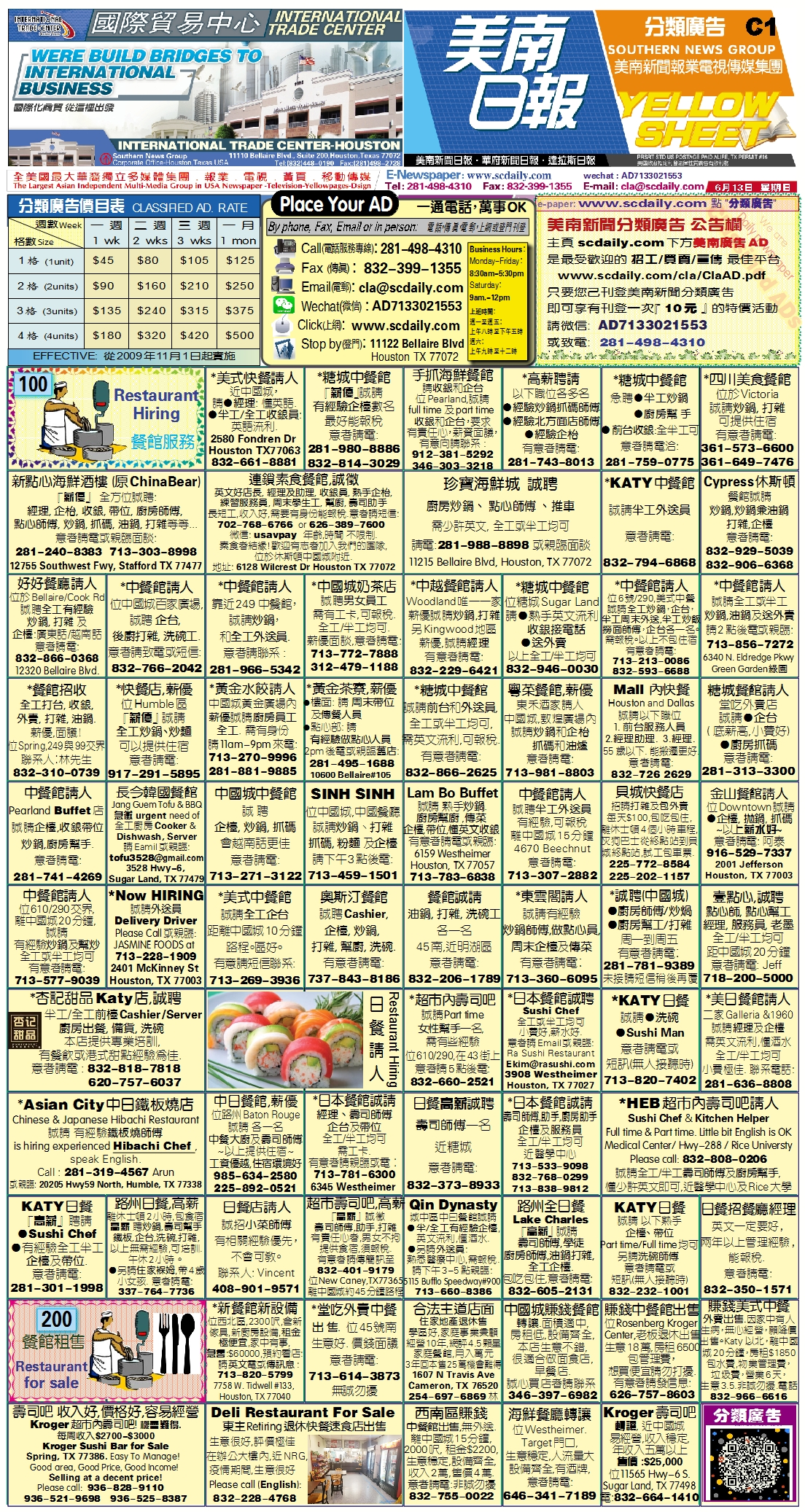210611 Epaper

 A Section B SectionC Section D Section